# NCERT Exemplar Solutions for Class 7 Maths Chapter 1 Integers

NCERT Exemplar Solutions for Class 7 Maths Chapter 1 Integers is the best study material for those students who are finding difficulties in solving problems. These solutions can help students clear doubts quickly and help in understanding the topic effectively. Our expert tutors formulate these exercises to assist you with your exam preparation to attain good marks in the subject. Students who wish to score good marks in Maths practise NCERT Exemplar Solutions for Class 7 Maths.

Chapter 1 – Integers solutions are available for download in pdf format and provide solutions to all the questions provided in NCERT Exemplar Class 7 Maths Chapter 1. An Integer is a whole number that can be positive, negative or zero. Positive integers are used in many ways during daily life. Highway numbers are posted along with roadway speed limits. Negative integers are used in thermometer readings, keeping scores in some games, etc. Now, let us have a look at some of the concepts discussed in this chapter.

• Representation of integers on the number line and their addition

and subtraction.

• Properties of Addition and Subtraction of Integers
• Multiplication of Integers
• Multiplication of a Positive and Negative Integer
• Division of a Positive and Negative Integer.

## Download the PDF of NCERT Exemplar Solutions for Class 7 Maths Chapter 1 Integers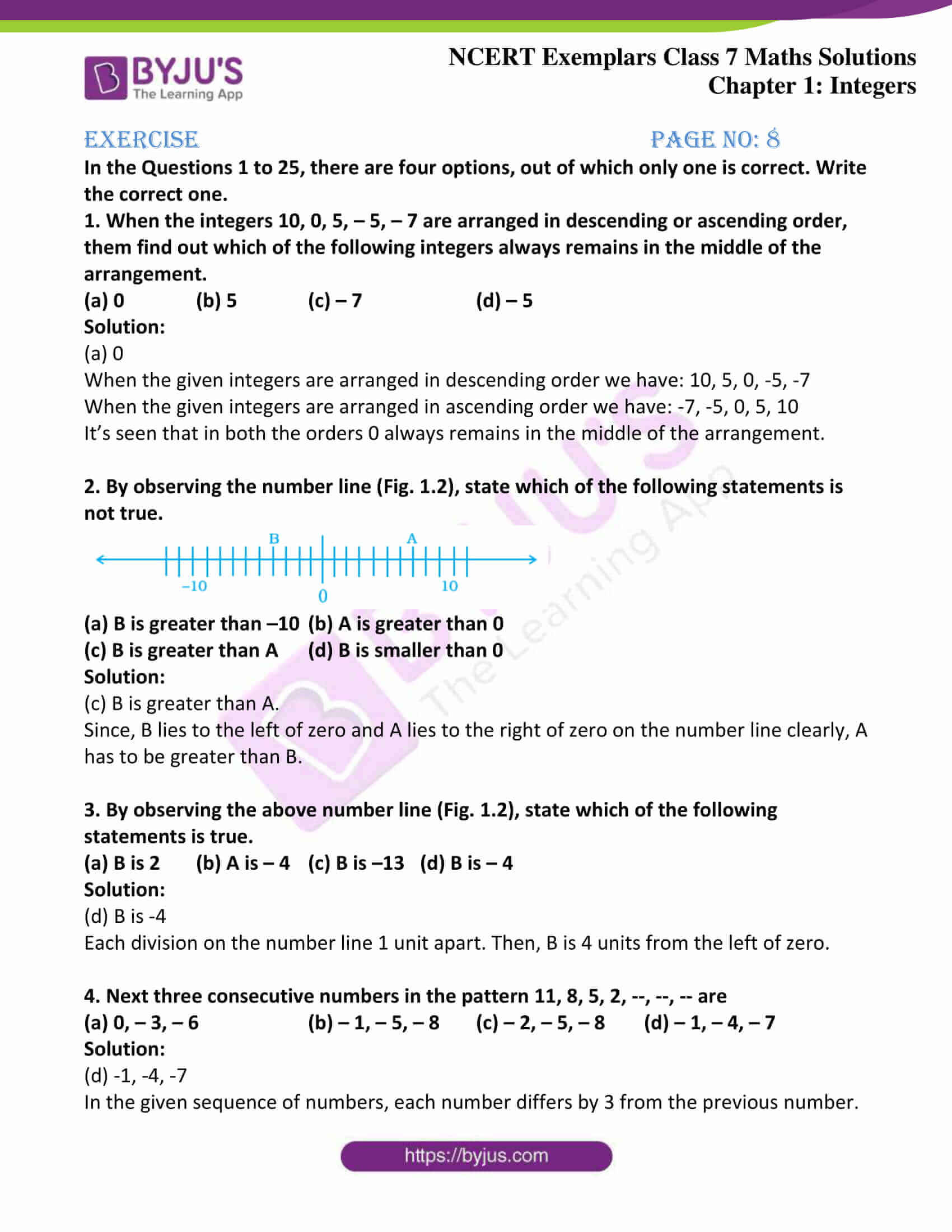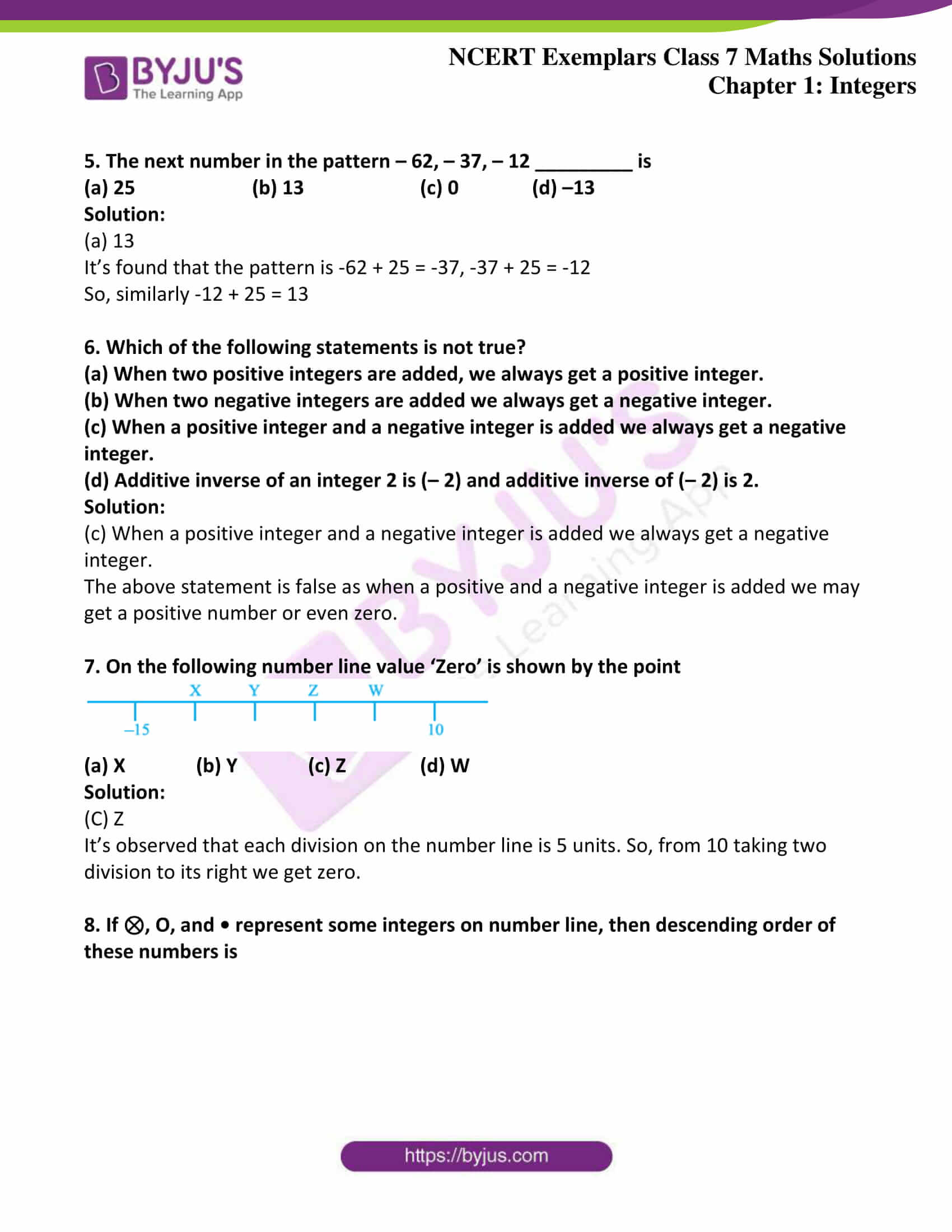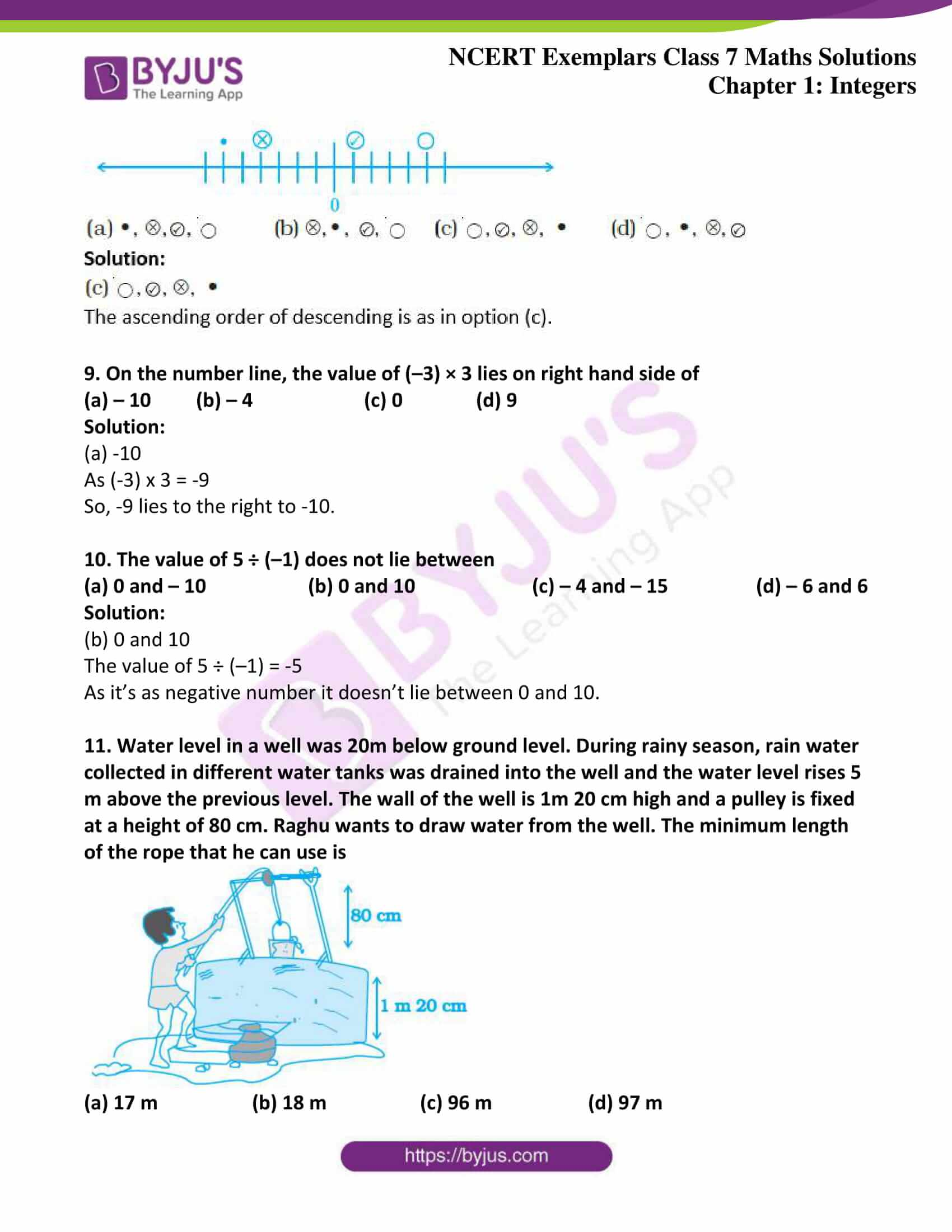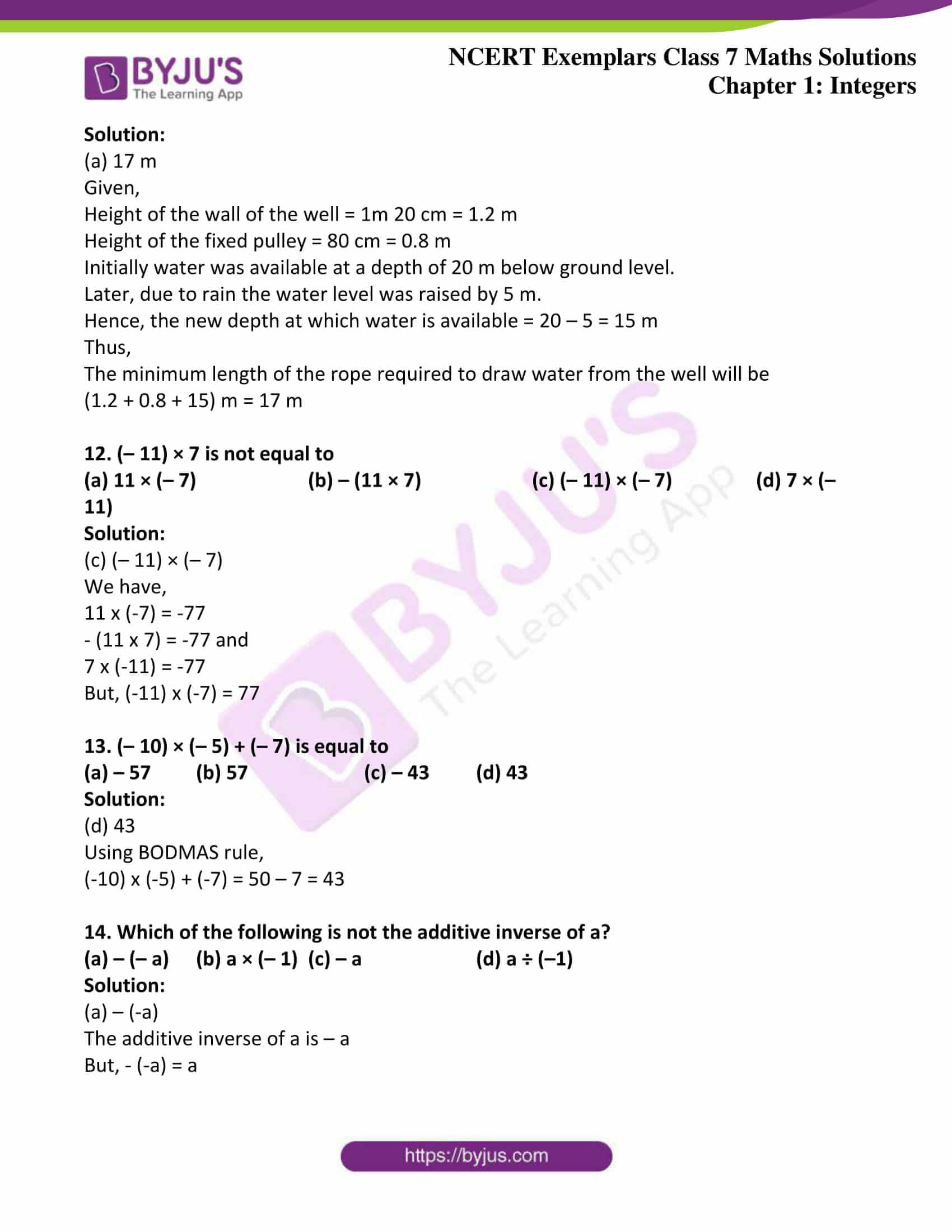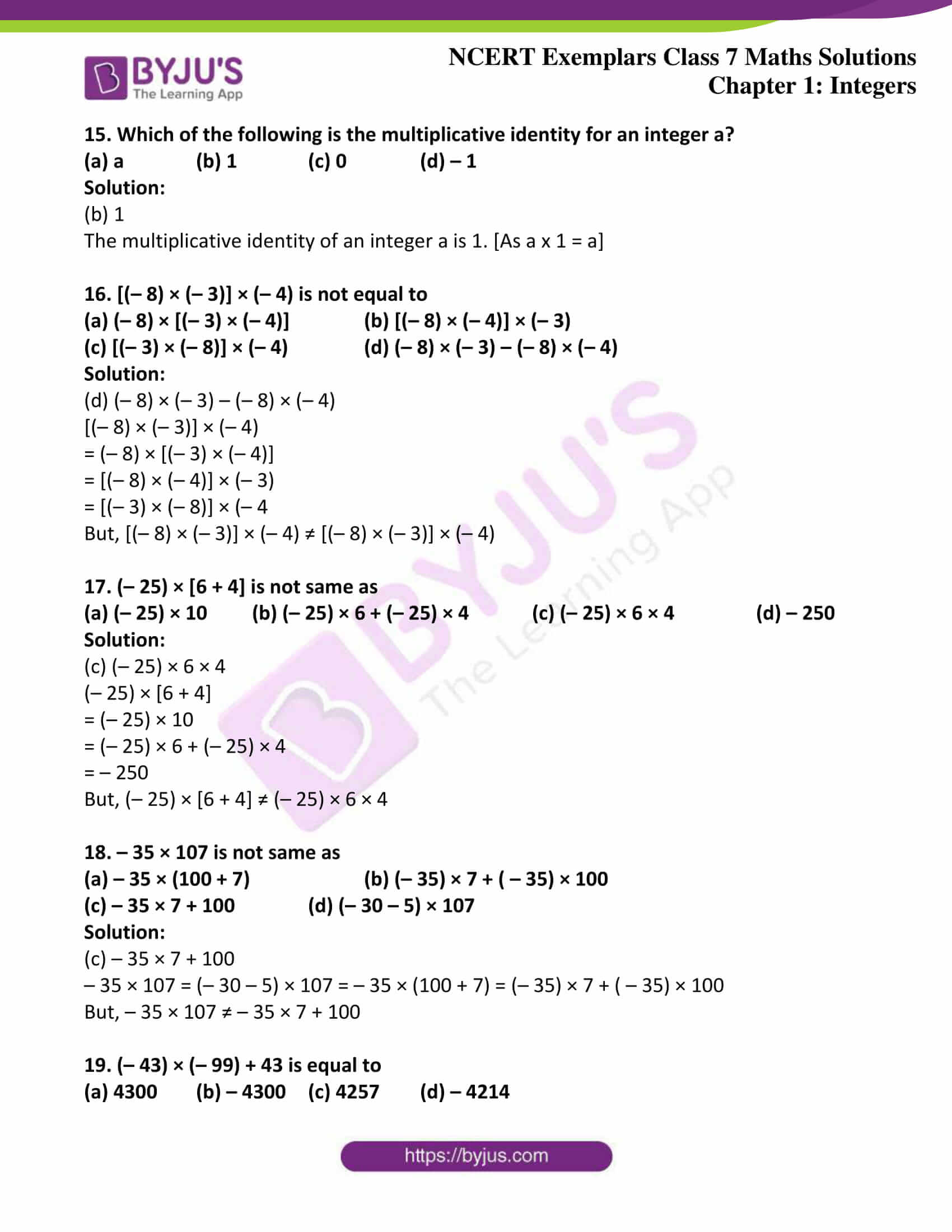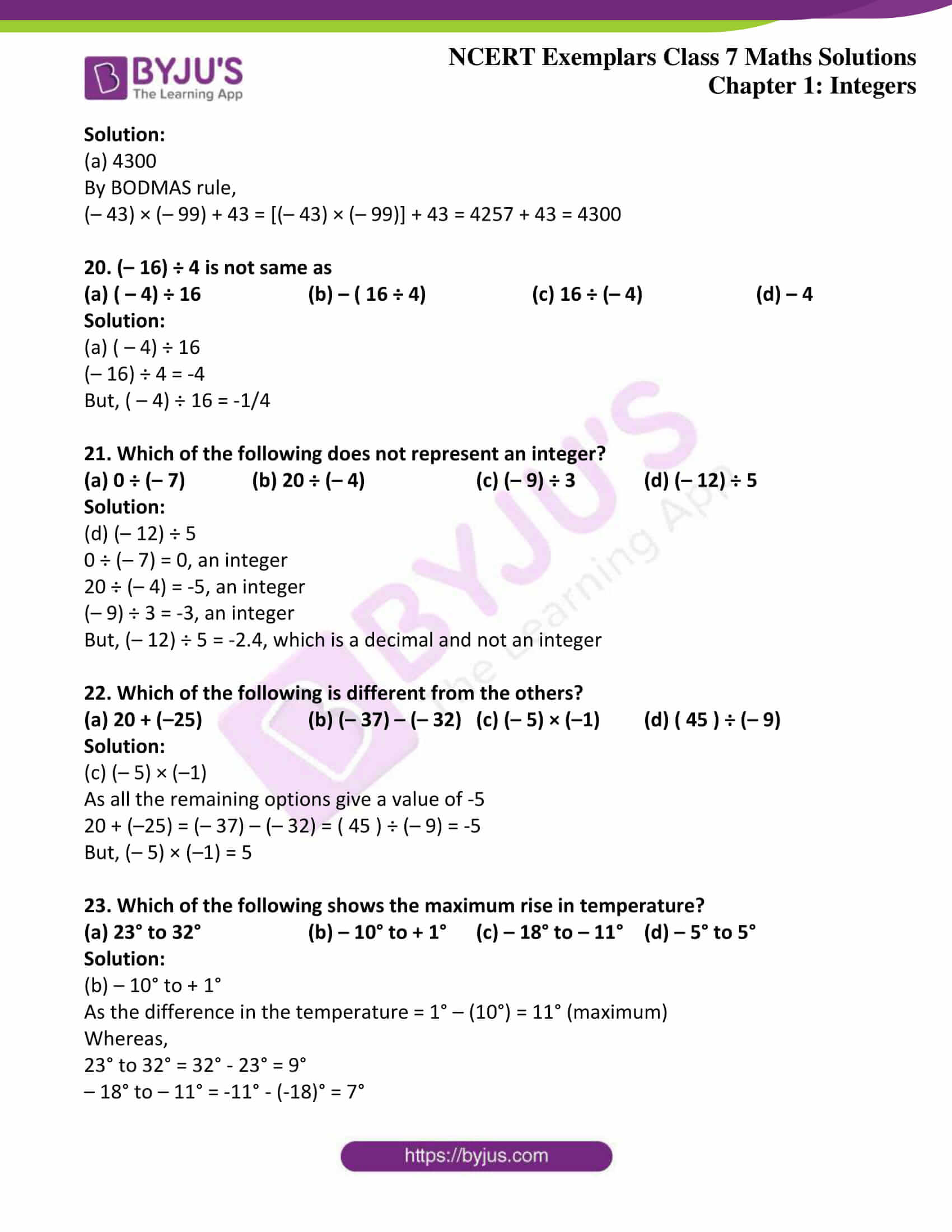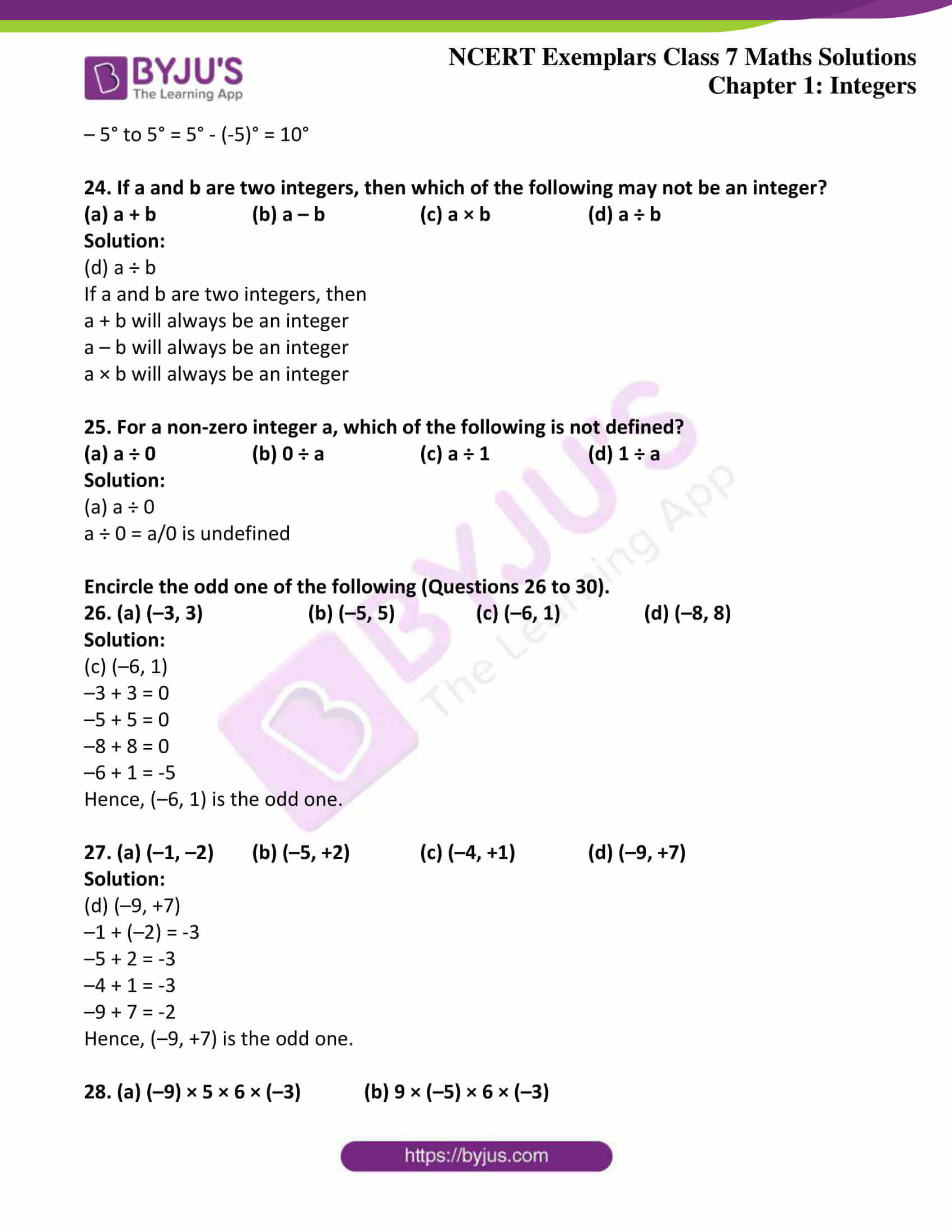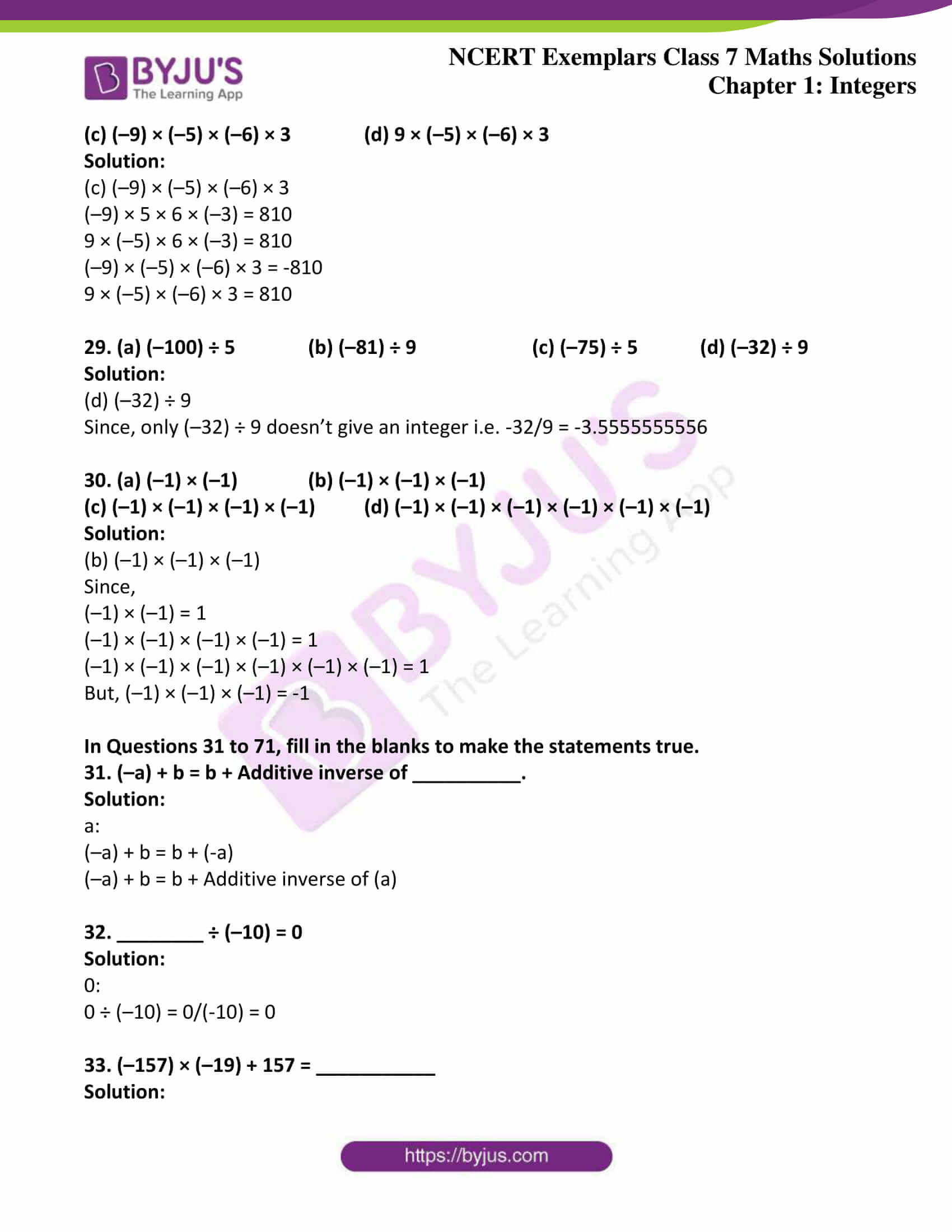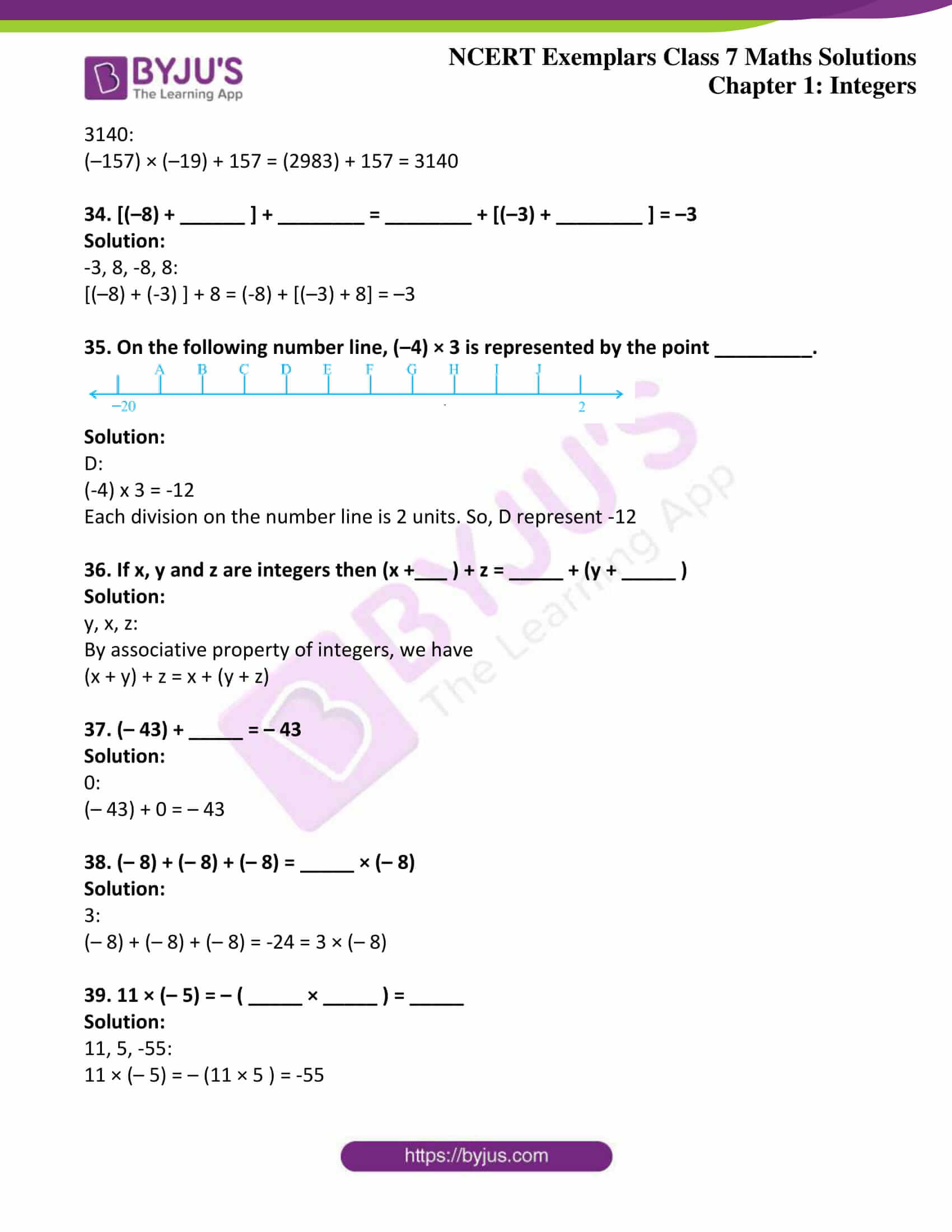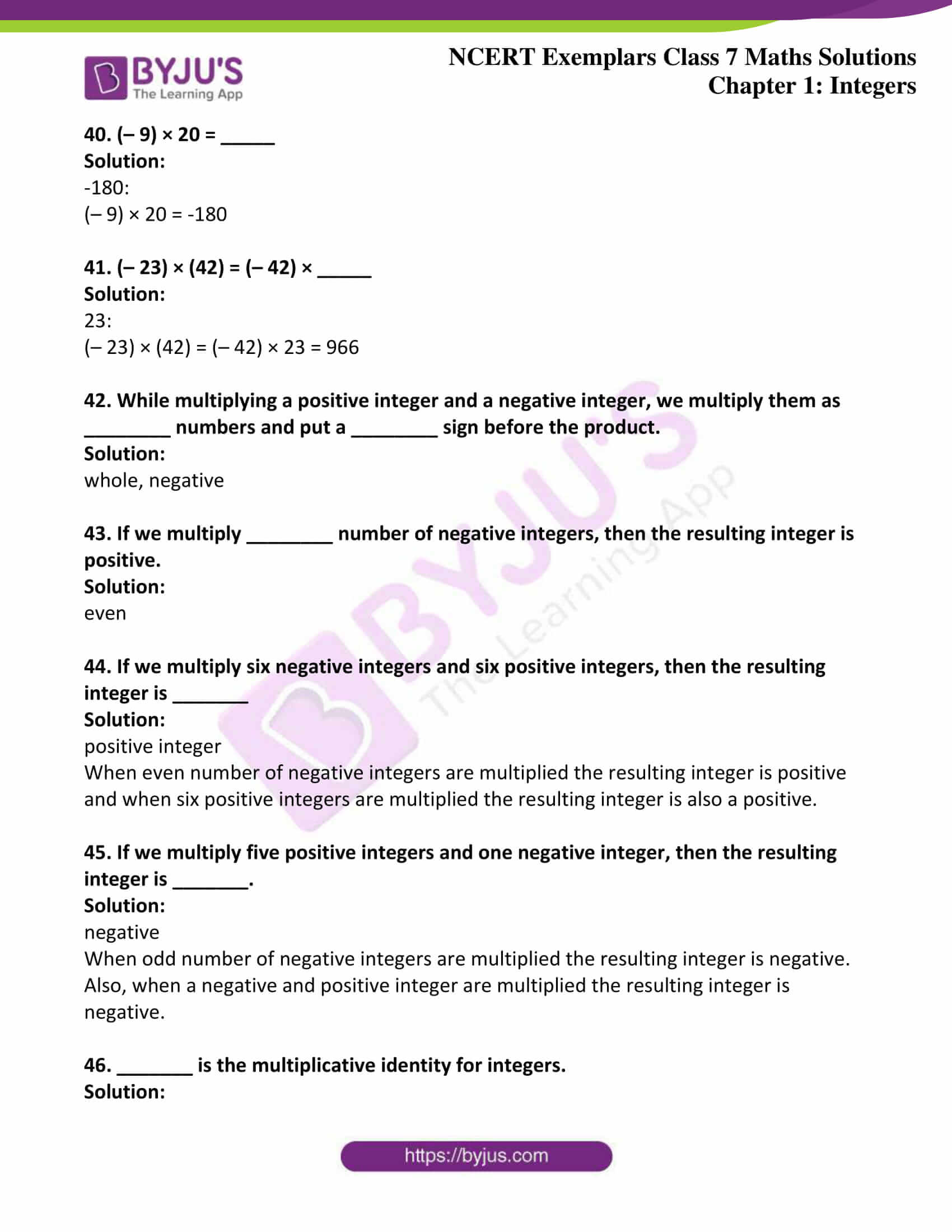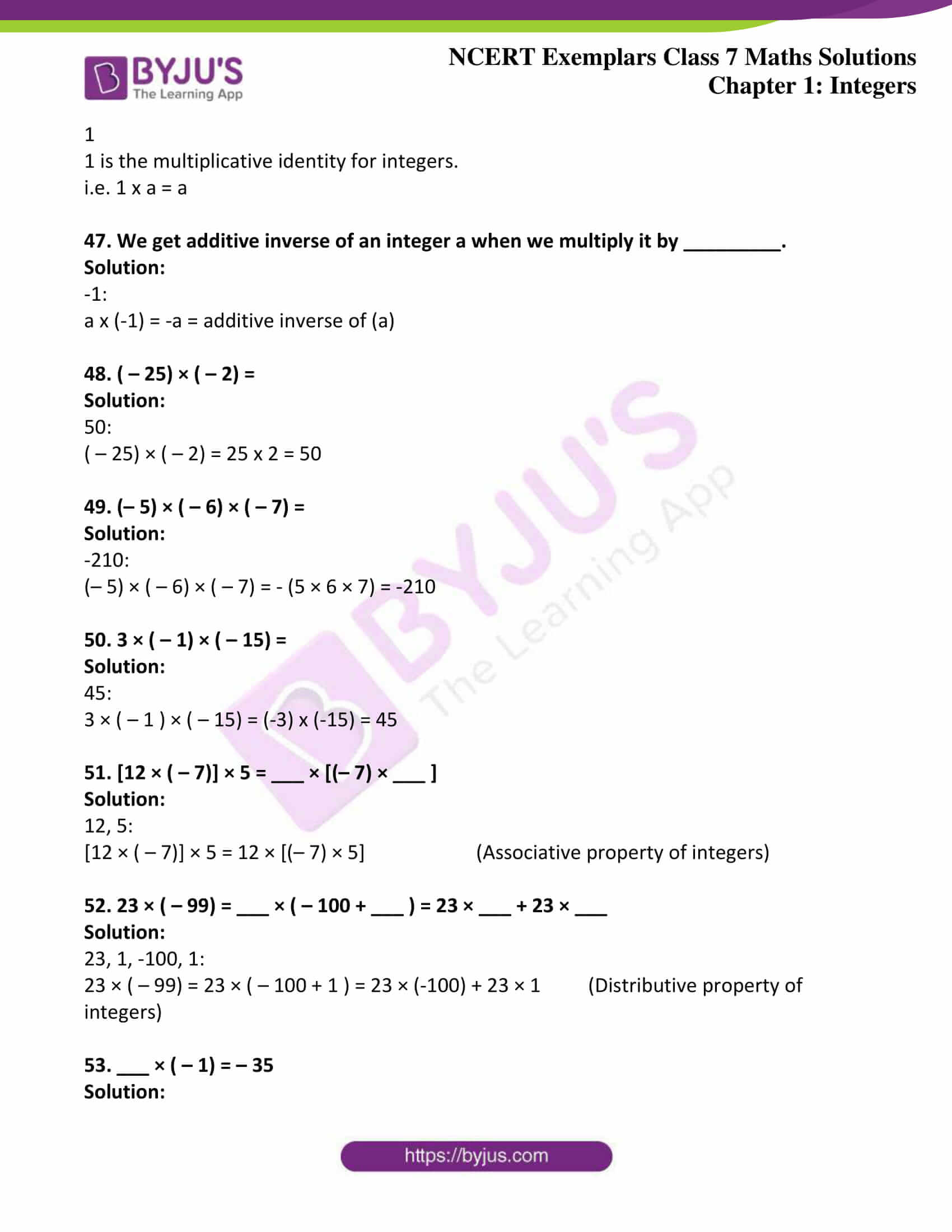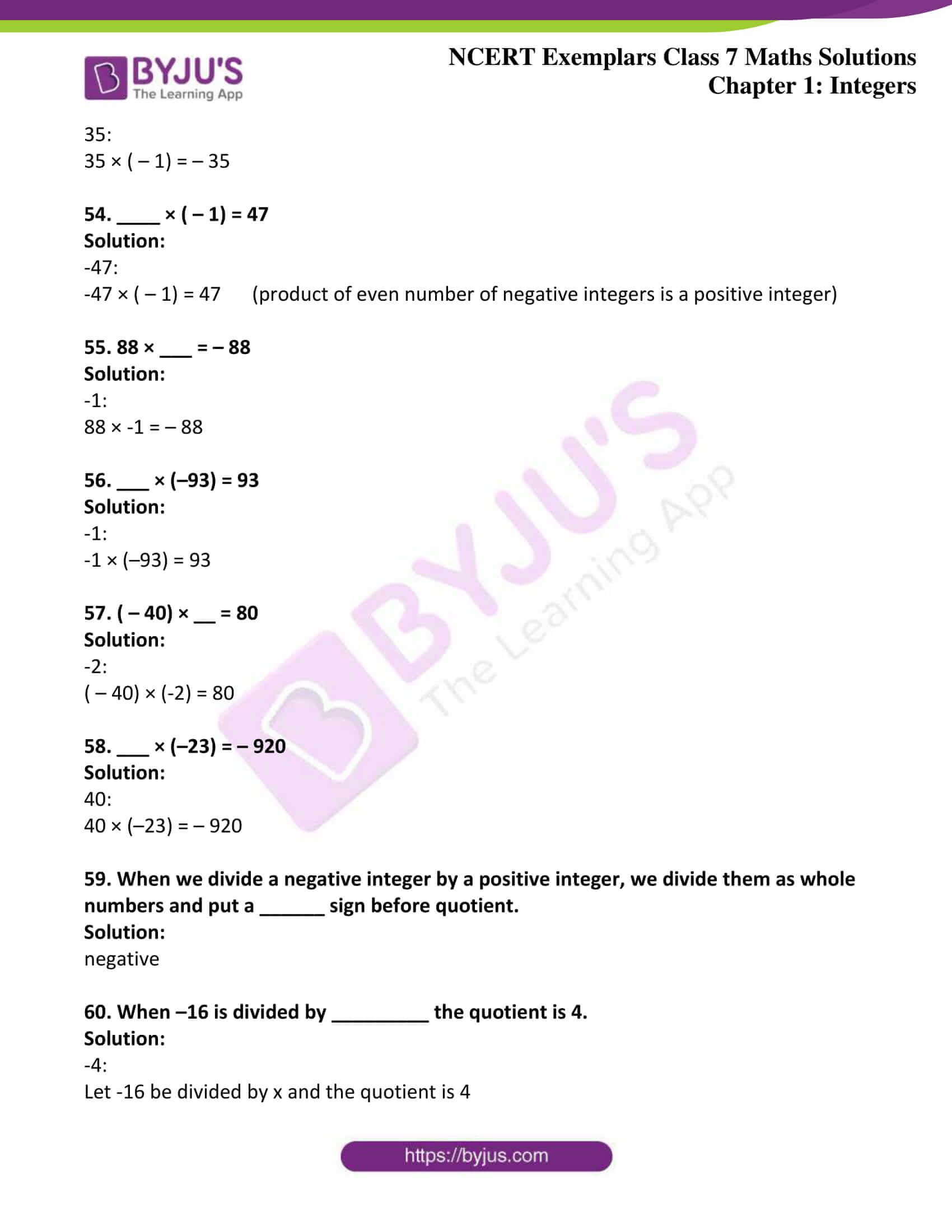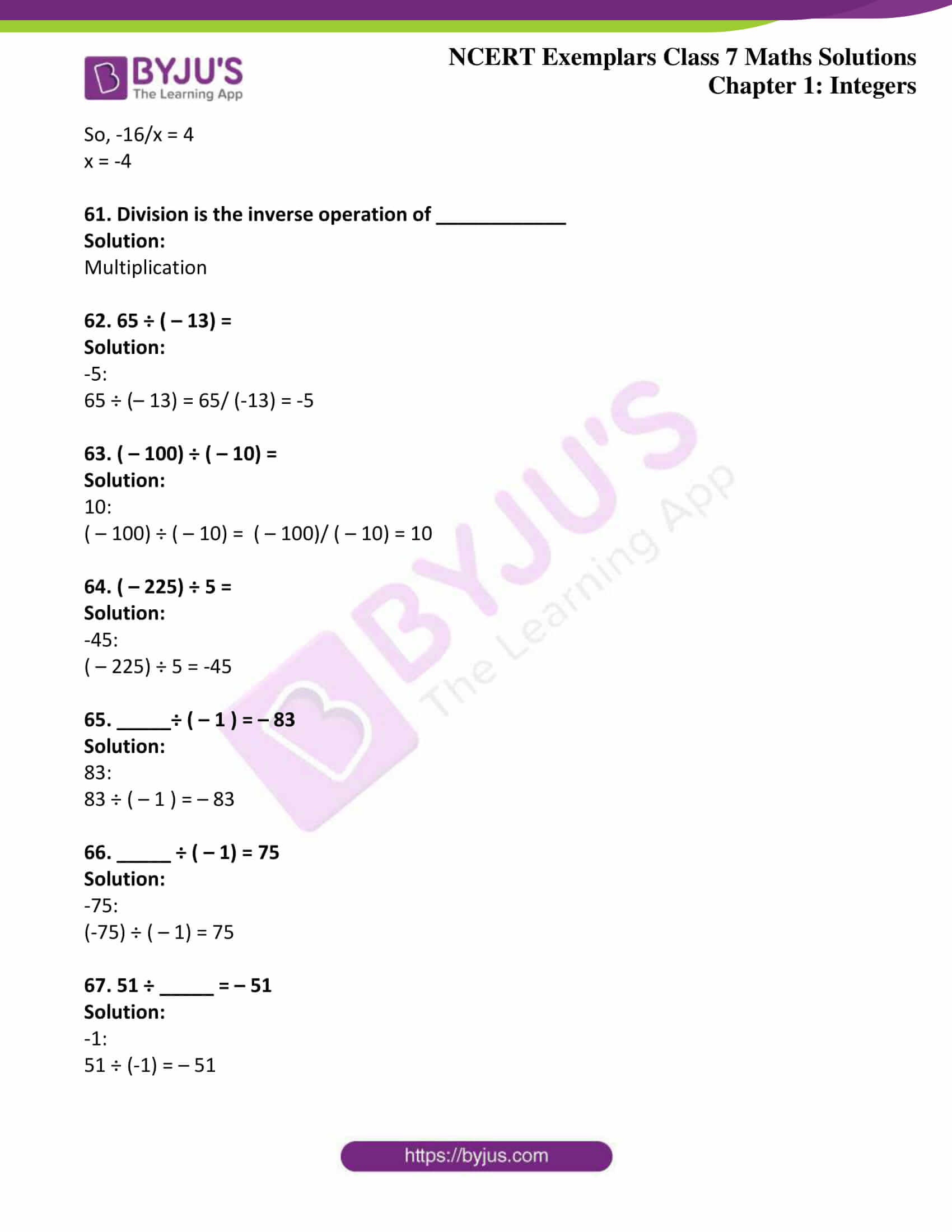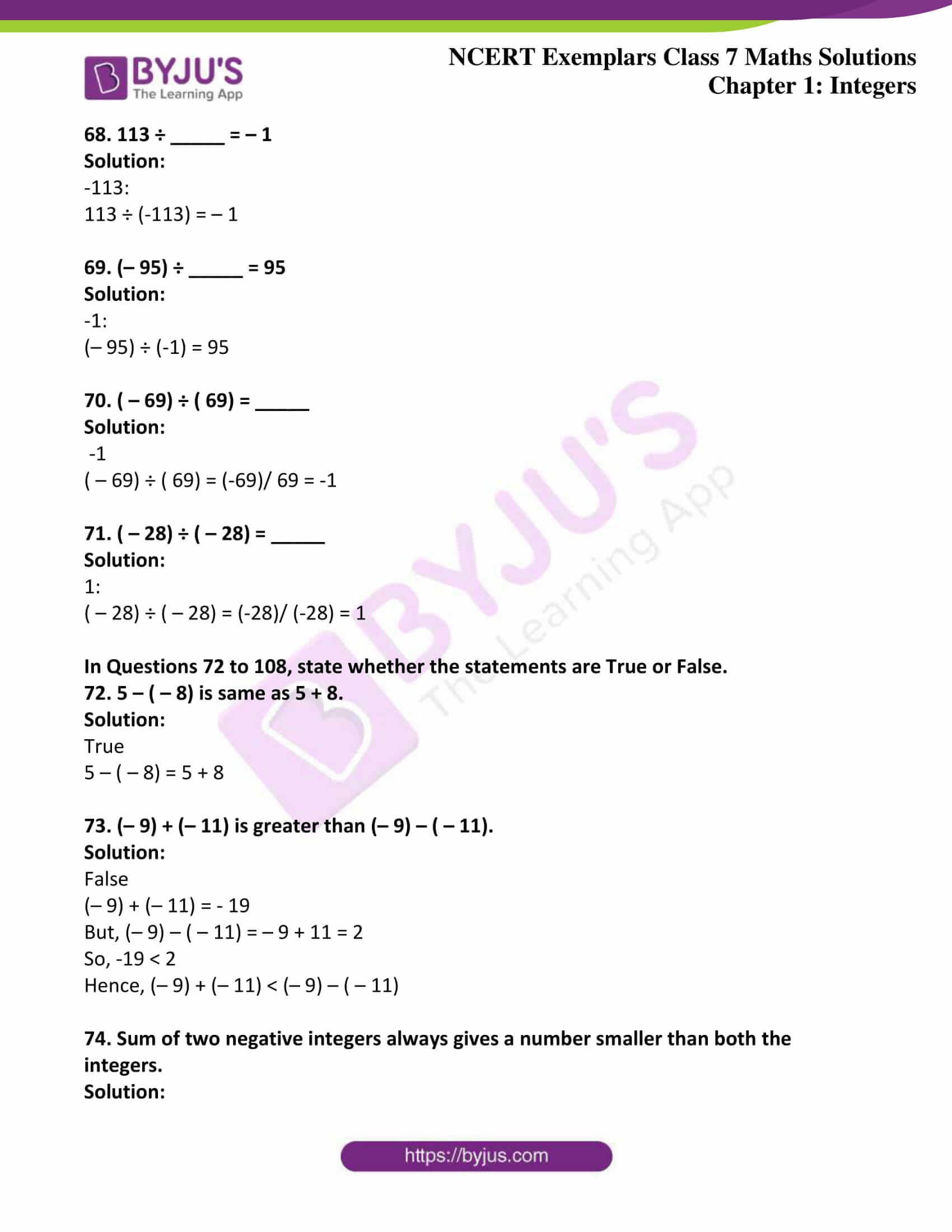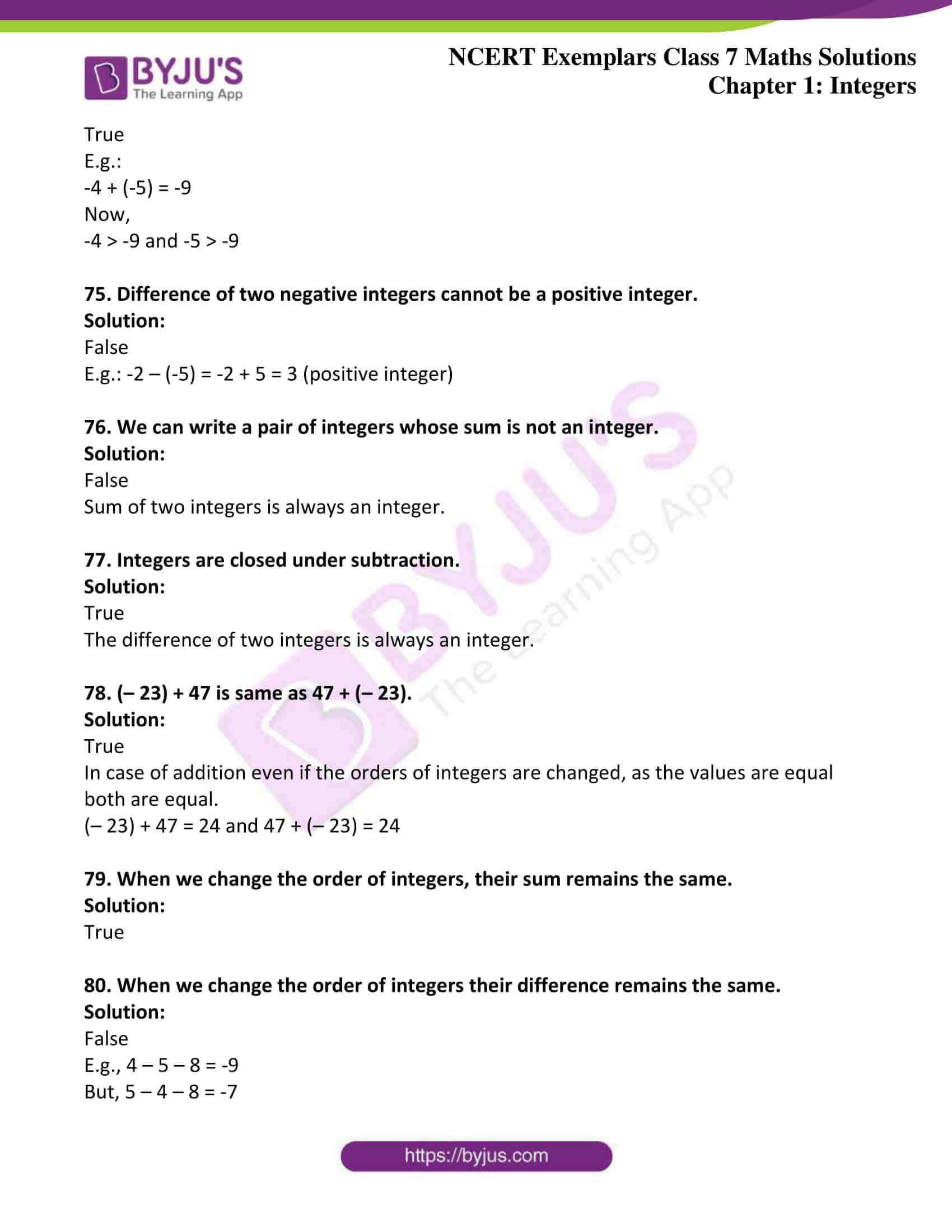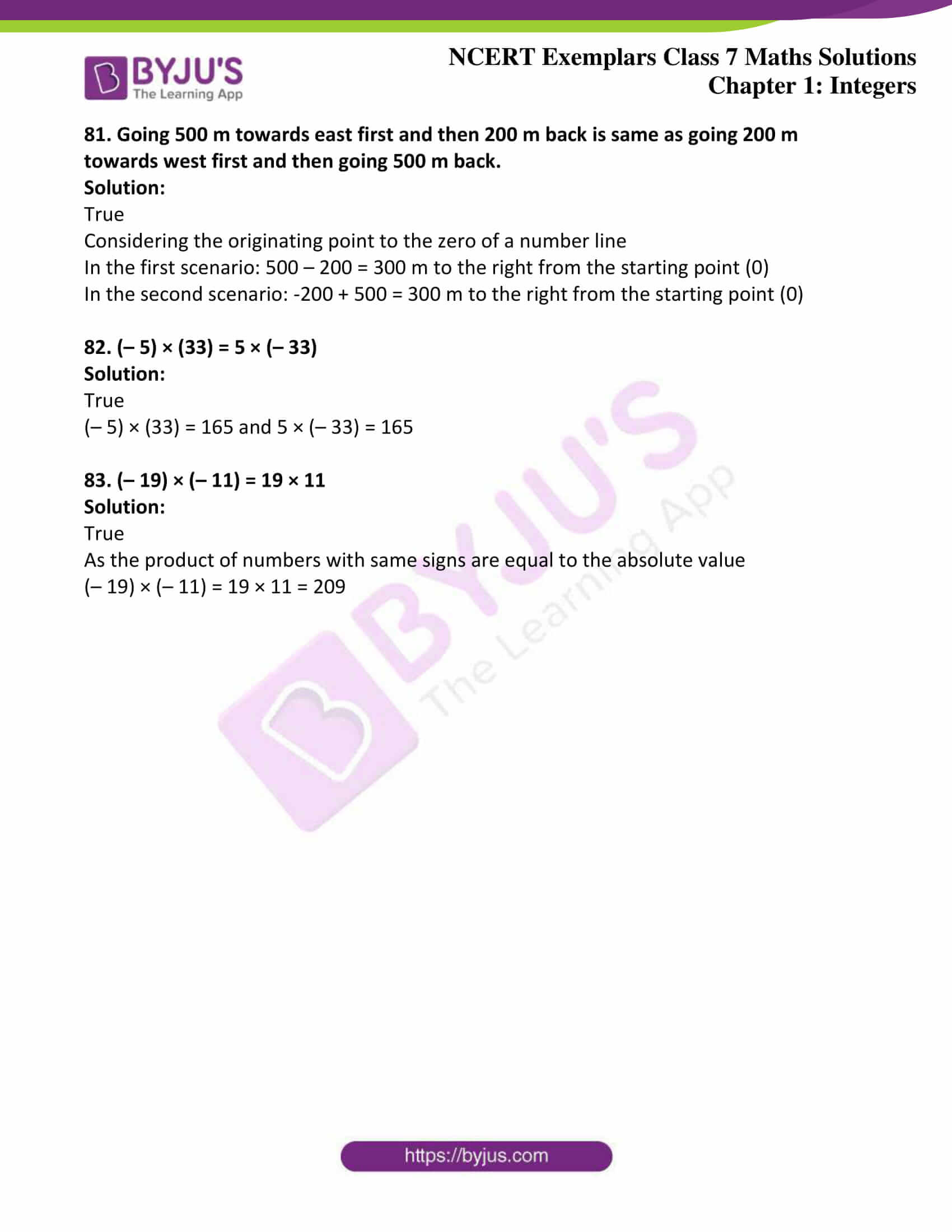### Access answers to Maths NCERT Exemplar Solutions for Class 7 Maths Chapter 1 Integers

Exercise Page No: 8

In the Questions 1 to 25, there are four options, out of which only one is correct. Write the correct one.

1. When the integers 10, 0, 5, – 5, – 7 are arranged in descending or ascending order, them find out which of the following integers always remains in the middle of the arrangement.

(a) 0 (b) 5 (c) – 7 (d) – 5

Solution:

(a) 0

When the given integers are arranged in descending order we have: 10, 5, 0, -5, -7

When the given integers are arranged in ascending order we have: -7, -5, 0, 5, 10

It’s seen that in both the orders 0 always remains in the middle of the arrangement.

2. By observing the number line (Fig. 1.2), state which of the following statements is not true.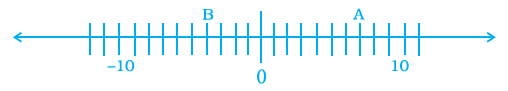(a) B is greater than –10 (b) A is greater than 0

(c) B is greater than A (d) B is smaller than 0

Solution:

(c) B is greater than A.

Since, B lies to the left of zero and A lies to the right of zero on the number line clearly, A has to be greater than B.

3. By observing the above number line (Fig. 1.2), state which of the following statements is true.

(a) B is 2 (b) A is – 4 (c) B is –13 (d) B is – 4

Solution:

(d) B is -4

Each division on the number line 1 unit apart. Then, B is 4 units from the left of zero.

4. Next three consecutive numbers in the pattern 11, 8, 5, 2, –, –, — are

(a) 0, – 3, – 6 (b) – 1, – 5, – 8 (c) – 2, – 5, – 8 (d) – 1, – 4, – 7

Solution:

(d) -1, -4, -7

In the given sequence of numbers, each number differs by 3 from the previous number.

5. The next number in the pattern – 62, – 37, – 12 _________ is

(a) 25 (b) 13 (c) 0 (d) –13

Solution:

(a) 13

It’s found that the pattern is -62 + 25 = -37, -37 + 25 = -12

So, similarly -12 + 25 = 13

6. Which of the following statements is not true?

(a) When two positive integers are added, we always get a positive integer.

(b) When two negative integers are added we always get a negative integer.

(c) When a positive integer and a negative integer is added we always get a negative integer.

(d) Additive inverse of an integer 2 is (– 2) and additive inverse of (– 2) is 2.

Solution:

(c) When a positive integer and a negative integer is added we always get a negative integer.

The above statement is false as when a positive and a negative integer is added we may get a positive number or even zero.

7. On the following number line value ‘Zero’ is shown by the point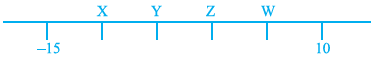(a) X (b) Y (c) Z (d) W

Solution:

(C) Z

It’s observed that each division on the number line is 5 units. So, from 10 taking two division to its right we get zero.

8. If ⊗, O, and • represent some integers on number line, then descending order of these numbers is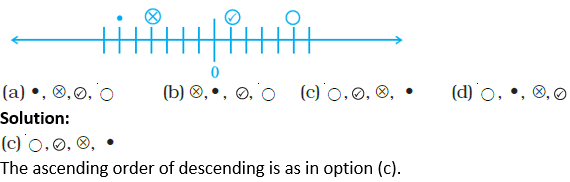9. On the number line, the value of (–3) × 3 lies on right hand side of

(a) – 10 (b) – 4 (c) 0 (d) 9

Solution:

(a) -10

As (-3) x 3 = -9

So, -9 lies to the right to -10.

10. The value of 5 ÷ (–1) does not lie between

(a) 0 and – 10 (b) 0 and 10 (c) – 4 and – 15 (d) – 6 and 6

Solution:

(b) 0 and 10

The value of 5 ÷ (–1) = -5

As it’s as negative number it doesn’t lie between 0 and 10.

11. Water level in a well was 20m below ground level. During rainy season, rain water collected in different water tanks was drained into the well and the water level rises 5 m above the previous level. The wall of the well is 1m 20 cm high and a pulley is fixed at a height of 80 cm. Raghu wants to draw water from the well. The minimum length of the rope that he can use is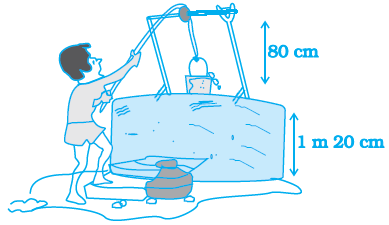(a) 17 m (b) 18 m (c) 96 m (d) 97 m

Solution:

(a) 17 m

Given,

Height of the wall of the well = 1m 20 cm = 1.2 m

Height of the fixed pulley = 80 cm = 0.8 m

Initially water was available at a depth of 20 m below ground level.

Later, due to rain the water level was raised by 5 m.

Hence, the new depth at which water is available = 20 – 5 = 15 m

Thus,

The minimum length of the rope required to draw water from the well will be

(1.2 + 0.8 + 15) m = 17 m

12. (– 11) × 7 is not equal to

(a) 11 × (– 7) (b) – (11 × 7) (c) (– 11) × (– 7) (d) 7 × (– 11)

Solution:

(c) (– 11) × (– 7)

We have,

11 x (-7) = -77

– (11 x 7) = -77 and

7 x (-11) = -77

But, (-11) x (-7) = 77

13. (– 10) × (– 5) + (– 7) is equal to

(a) – 57 (b) 57 (c) – 43 (d) 43

Solution:

(d) 43

Using BODMAS rule,

(-10) x (-5) + (-7) = 50 – 7 = 43

14. Which of the following is not the additive inverse of a?

(a) – (– a) (b) a × (– 1) (c) – a (d) a ÷ (–1)

Solution:

(a) – (-a)

The additive inverse of a is – a

But, – (-a) = a

15. Which of the following is the multiplicative identity for an integer a?

(a) a (b) 1 (c) 0 (d) – 1

Solution:

(b) 1

The multiplicative identity of an integer a is 1. [As a x 1 = a]

16. [(– 8) × (– 3)] × (– 4) is not equal to

(a) (– 8) × [(– 3) × (– 4)] (b) [(– 8) × (– 4)] × (– 3)

(c) [(– 3) × (– 8)] × (– 4) (d) (– 8) × (– 3) – (– 8) × (– 4)

Solution:

(d) (– 8) × (– 3) – (– 8) × (– 4)

[(– 8) × (– 3)] × (– 4)

= (– 8) × [(– 3) × (– 4)]

= [(– 8) × (– 4)] × (– 3)

= [(– 3) × (– 8)] × (– 4

But, [(– 8) × (– 3)] × (– 4) ≠ [(– 8) × (– 3)] × (– 4)

17. (– 25) × [6 + 4] is not same as

(a) (– 25) × 10 (b) (– 25) × 6 + (– 25) × 4 (c) (– 25) × 6 × 4 (d) – 250

Solution:

(c) (– 25) × 6 × 4

(– 25) × [6 + 4]

= (– 25) × 10

= (– 25) × 6 + (– 25) × 4

= – 250

But, (– 25) × [6 + 4] ≠ (– 25) × 6 × 4

18. – 35 × 107 is not same as

(a) – 35 × (100 + 7) (b) (– 35) × 7 + ( – 35) × 100

(c) – 35 × 7 + 100 (d) (– 30 – 5) × 107

Solution:

(c) – 35 × 7 + 100

– 35 × 107 = (– 30 – 5) × 107 = – 35 × (100 + 7) = (– 35) × 7 + ( – 35) × 100

But, – 35 × 107 ≠ – 35 × 7 + 100

19. (– 43) × (– 99) + 43 is equal to

(a) 4300 (b) – 4300 (c) 4257 (d) – 4214

Solution:

(a) 4300

By BODMAS rule,

(– 43) × (– 99) + 43 = [(– 43) × (– 99)] + 43 = 4257 + 43 = 4300

20. (– 16) ÷ 4 is not same as

(a) ( – 4) ÷ 16 (b) – ( 16 ÷ 4) (c) 16 ÷ (– 4) (d) – 4

Solution:

(a) ( – 4) ÷ 16

(– 16) ÷ 4 = -4

But, ( – 4) ÷ 16 = -1/4

21. Which of the following does not represent an integer?

(a) 0 ÷ (– 7) (b) 20 ÷ (– 4) (c) (– 9) ÷ 3 (d) (– 12) ÷ 5

Solution:

(d) (– 12) ÷ 5

0 ÷ (– 7) = 0, an integer

20 ÷ (– 4) = -5, an integer

(– 9) ÷ 3 = -3, an integer

But, (– 12) ÷ 5 = -2.4, which is a decimal and not an integer

22. Which of the following is different from the others?

(a) 20 + (–25) (b) (– 37) – (– 32) (c) (– 5) × (–1) (d) ( 45 ) ÷ (– 9)

Solution:

(c) (– 5) × (–1)

As all the remaining options give a value of -5

20 + (–25) = (– 37) – (– 32) = ( 45 ) ÷ (– 9) = -5

But, (– 5) × (–1) = 5

23. Which of the following shows the maximum rise in temperature?

(a) 23° to 32° (b) – 10° to + 1° (c) – 18° to – 11° (d) – 5° to 5°

Solution:

(b) – 10° to + 1°

As the difference in the temperature = 1° – (10°) = 11° (maximum)

Whereas,

23° to 32° = 32° – 23° = 9°

– 18° to – 11° = -11° – (-18)° = 7°

– 5° to 5° = 5° – (-5)° = 10°

24. If a and b are two integers, then which of the following may not be an integer?

(a) a + b (b) a – b (c) a × b (d) a ÷ b

Solution:

(d) a ÷ b

If a and b are two integers, then

a + b will always be an integer

a – b will always be an integer

a × b will always be an integer

25. For a non-zero integer a, which of the following is not defined?

(a) a ÷ 0 (b) 0 ÷ a (c) a ÷ 1 (d) 1 ÷ a

Solution:

(a) a ÷ 0

a ÷ 0 = a/0 is undefined

Encircle the odd one of the following (Questions 26 to 30).

26. (a) (–3, 3) (b) (–5, 5) (c) (–6, 1) (d) (–8, 8)

Solution:

(c) (–6, 1)

–3 + 3 = 0

–5 + 5 = 0

–8 + 8 = 0

–6 + 1 = -5

Hence, (–6, 1) is the odd one.

27. (a) (–1, –2) (b) (–5, +2) (c) (–4, +1) (d) (–9, +7)

Solution:

(d) (–9, +7)

–1 + (–2) = -3

–5 + 2 = -3

–4 + 1 = -3

–9 + 7 = -2

Hence, (–9, +7) is the odd one.

28. (a) (–9) × 5 × 6 × (–3) (b) 9 × (–5) × 6 × (–3)

(c) (–9) × (–5) × (–6) × 3 (d) 9 × (–5) × (–6) × 3

Solution:

(c) (–9) × (–5) × (–6) × 3

(–9) × 5 × 6 × (–3) = 810

9 × (–5) × 6 × (–3) = 810

(–9) × (–5) × (–6) × 3 = -810

9 × (–5) × (–6) × 3 = 810

29. (a) (–100) ÷ 5 (b) (–81) ÷ 9 (c) (–75) ÷ 5 (d) (–32) ÷ 9

Solution:

(d) (–32) ÷ 9

Since, only (–32) ÷ 9 doesn’t give an integer i.e. -32/9 = -3.5555555556

30. (a) (–1) × (–1) (b) (–1) × (–1) × (–1)

(c) (–1) × (–1) × (–1) × (–1) (d) (–1) × (–1) × (–1) × (–1) × (–1) × (–1)

Solution:

(b) (–1) × (–1) × (–1)

Since,

(–1) × (–1) = 1

(–1) × (–1) × (–1) × (–1) = 1

(–1) × (–1) × (–1) × (–1) × (–1) × (–1) = 1

But, (–1) × (–1) × (–1) = -1

In Questions 31 to 71, fill in the blanks to make the statements true.

31. (–a) + b = b + Additive inverse of __________.

Solution:

a:

(–a) + b = b + (-a)

(–a) + b = b + Additive inverse of (a)

32. ________ ÷ (–10) = 0

Solution:

0:

0 ÷ (–10) = 0/(-10) = 0

33. (–157) × (–19) + 157 = ___________

Solution:

3140:

(–157) × (–19) + 157 = (2983) + 157 = 3140

34. [(–8) + ______ ] + ________ = ________ + [(–3) + ________ ] = –3

Solution:

-3, 8, -8, 8:

[(–8) + (-3) ] + 8 = (-8) + [(–3) + 8] = –3

35. On the following number line, (–4) × 3 is represented by the point _________.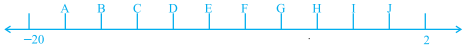Solution:

D:

(-4) x 3 = -12

Each division on the number line is 2 units. So, D represent -12

36. If x, y and z are integers then (x +___ ) + z = _____ + (y + _____ )

Solution:

y, x, z:

By associative property of integers, we have

(x + y) + z = x + (y + z)

37. (– 43) + _____ = – 43

Solution:

0:

(– 43) + 0 = – 43

38. (– 8) + (– 8) + (– 8) = _____ × (– 8)

Solution:

3:

(– 8) + (– 8) + (– 8) = -24 = 3 × (– 8)

39. 11 × (– 5) = – ( _____ × _____ ) = _____

Solution:

11, 5, -55:

11 × (– 5) = – (11 × 5 ) = -55

40. (– 9) × 20 = _____

Solution:

-180:

(– 9) × 20 = -180

41. (– 23) × (42) = (– 42) × _____

Solution:

23:

(– 23) × (42) = (– 42) × 23 = 966

42. While multiplying a positive integer and a negative integer, we multiply them as ________ numbers and put a ________ sign before the product.

Solution:

whole, negative

43. If we multiply ________ number of negative integers, then the resulting integer is positive.

Solution:

even

44. If we multiply six negative integers and six positive integers, then the resulting integer is _______

Solution:

positive integer

When even number of negative integers are multiplied the resulting integer is positive and when six positive integers are multiplied the resulting integer is also a positive.

45. If we multiply five positive integers and one negative integer, then the resulting integer is _______.

Solution:

negative

When odd number of negative integers are multiplied the resulting integer is negative. Also, when a negative and positive integer are multiplied the resulting integer is negative.

46. _______ is the multiplicative identity for integers.

Solution:

1

1 is the multiplicative identity for integers.

i.e. 1 x a = a

47. We get additive inverse of an integer a when we multiply it by _________.

Solution:

-1:

a x (-1) = -a = additive inverse of (a)

48. ( – 25) × ( – 2) =

Solution:

50:

( – 25) × ( – 2) = 25 x 2 = 50

49. (– 5) × ( – 6) × ( – 7) =

Solution:

-210:

(– 5) × ( – 6) × ( – 7) = – (5 × 6 × 7) = -210

50. 3 × ( – 1) × ( – 15) =

Solution:

45:

3 × ( – 1 ) × ( – 15) = (-3) x (-15) = 45

51. [12 × ( – 7)] × 5 = ___ × [(– 7) × ___ ]

Solution:

12, 5:

[12 × ( – 7)] × 5 = 12 × [(– 7) × 5] (Associative property of integers)

52. 23 × ( – 99) = ___ × ( – 100 + ___ ) = 23 × ___ + 23 × ___

Solution:

23, 1, -100, 1:

23 × ( – 99) = 23 × ( – 100 + 1 ) = 23 × (-100) + 23 × 1 (Distributive property of integers)

53. ___ × ( – 1) = – 35

Solution:

35:

35 × ( – 1) = – 35

54. ____ × ( – 1) = 47

Solution:

-47:

-47 × ( – 1) = 47 (product of even number of negative integers is a positive integer)

55. 88 × ___ = – 88

Solution:

-1:

88 × -1 = – 88

56. ___ × (–93) = 93

Solution:

-1:

-1 × (–93) = 93

57. ( – 40) × __ = 80

Solution:

-2:

( – 40) × (-2) = 80

58. ___ × (–23) = – 920

Solution:

40:

40 × (–23) = – 920

59. When we divide a negative integer by a positive integer, we divide them as whole numbers and put a ______ sign before quotient.

Solution:

negative

60. When –16 is divided by _________ the quotient is 4.

Solution:

-4:

Let -16 be divided by x and the quotient is 4

So, -16/x = 4

x = -4

61. Division is the inverse operation of ____________

Solution:

Multiplication

62. 65 ÷ ( – 13) =

Solution:

-5:

65 ÷ (– 13) = 65/ (-13) = -5

63. ( – 100) ÷ ( – 10) =

Solution:

10:

( – 100) ÷ ( – 10) = ( – 100)/ ( – 10) = 10

64. ( – 225) ÷ 5 =

Solution:

-45:

( – 225) ÷ 5 = -45

65. _____÷ ( – 1 ) = – 83

Solution:

83:

83 ÷ ( – 1 ) = – 83

66. _____ ÷ ( – 1) = 75

Solution:

-75:

(-75) ÷ ( – 1) = 75

67. 51 ÷ _____ = – 51

Solution:

-1:

51 ÷ (-1) = – 51

68. 113 ÷ _____ = – 1

Solution:

-113:

113 ÷ (-113) = – 1

69. (– 95) ÷ _____ = 95

Solution:

-1:

(– 95) ÷ (-1) = 95

70. ( – 69) ÷ ( 69) = _____

Solution:

-1

( – 69) ÷ ( 69) = (-69)/ 69 = -1

71. ( – 28) ÷ ( – 28) = _____

Solution:

1:

( – 28) ÷ ( – 28) = (-28)/ (-28) = 1

In Questions 72 to 108, state whether the statements are True or False.

72. 5 – ( – 8) is same as 5 + 8.

Solution:

True

5 – ( – 8) = 5 + 8

73. (– 9) + (– 11) is greater than (– 9) – ( – 11).

Solution:

False

(– 9) + (– 11) = – 19

But, (– 9) – ( – 11) = – 9 + 11 = 2

So, -19 < 2

Hence, (– 9) + (– 11) < (– 9) – ( – 11)

74. Sum of two negative integers always gives a number smaller than both the integers.

Solution:

True

E.g.:

-4 + (-5) = -9

Now,

-4 > -9 and -5 > -9

75. Difference of two negative integers cannot be a positive integer.

Solution:

False

E.g.: -2 – (-5) = -2 + 5 = 3 (positive integer)

76. We can write a pair of integers whose sum is not an integer.

Solution:

False

Sum of two integers is always an integer.

77. Integers are closed under subtraction.

Solution:

True

The difference of two integers is always an integer.

78. (– 23) + 47 is same as 47 + (– 23).

Solution:

True

In case of addition even if the orders of integers are changed, as the values are equal both are equal.

(– 23) + 47 = 24 and 47 + (– 23) = 24

79. When we change the order of integers, their sum remains the same.

Solution:

True

80. When we change the order of integers their difference remains the same.

Solution:

False

E.g., 4 – 5 – 8 = -9

But, 5 – 4 – 8 = -7

81. Going 500 m towards east first and then 200 m back is same as going 200 m towards west first and then going 500 m back.

Solution:

True

Considering the originating point to the zero of a number line

In the first scenario: 500 – 200 = 300 m to the right from the starting point (0)

In the second scenario: -200 + 500 = 300 m to the right from the starting point (0)

82. (– 5) × (33) = 5 × (– 33)

Solution:

True

(– 5) × (33) = 165 and 5 × (– 33) = 165

83. (– 19) × (– 11) = 19 × 11

Solution:

True

As the product of numbers with same signs are equal to the absolute value

(– 19) × (– 11) = 19 × 11 = 209